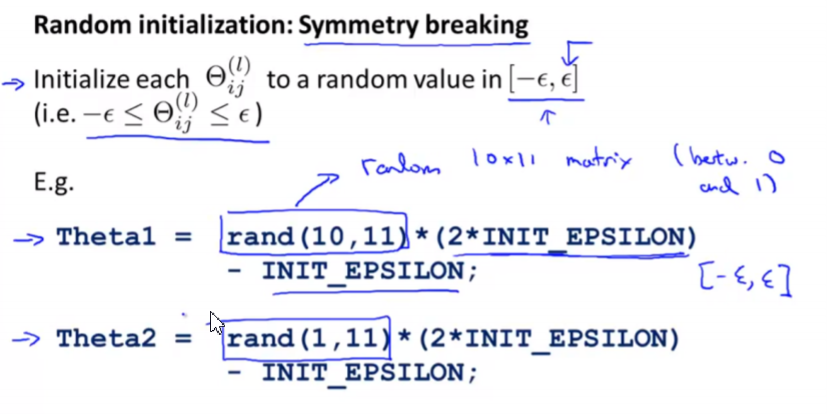Scott's world.

# Week1-Week5总结

Word count: 351Reading time: 2 min
2019/06/23 Share### Liner Regression

• Cost Function

$h(x)=\theta_0+\theta_1x+….$

$h(x)=\theta^Tx$

• Linear Regression

$J(\theta) = \frac{1}{2m}\sum{1}^{m}(h\theta(x^i)-y^i)$

$\frac{\partial{J(\theta)}}{\partial{\thetaj}}=\frac{1}{m}\sum{1}^{m}(h_\theta(x^i)-y^i)$

• Gradient descent algorithm

repeat until convergence{

$\thetaj := \theta_j - \frac{ \alpha}{m}\sum{i=1}^{m}(h_\theta(x^{(i)})-y^{(i)}) x^{(i)}$

}

• Feature scaling and mean normalization

$x_i=\frac{x_i-\mu_i}{s_i}$

$\mu_i$: the average of all the values for feature (i)

$s_i$ : standard deviation

• learning rate

If α is too small: slow convergence.
If α is too large: may not decrease on every iteration and thus may not converge.

• Polynomial Regression

change the behavior or curve of our hypothesis function by making it a quadratic, cubic or square root function (or any other form).

• Normal Equation

$\theta = (X^TX)^{-1}X^Ty$

### Logistic Regression

• Logistic Function or Sigmoid Function

![](https://raw.githubusercontent.com/catwithtudou/photo/master/20190618224141.png)

• Decision Boundary

$\theta^Tx \ge 0 \Rightarrow y=1$

$\theta^Tx \le 0 \Rightarrow y=0$

• Cost Function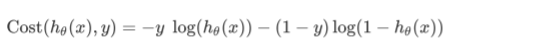• Gradient Descent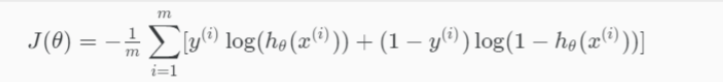• $h=g(X\theta)$

$J(\theta)=\frac{1}{m}(-y’log(h)-(1-y)’log(1-h))$

• $\theta:=\theta-\frac{\alpha}{m}X^T(g(\theta X) -y)$

• Advanced Optimization

• Multiclass Classification: One-vs-all

Train a logistic regression classifier $h\theta(X)$ for each class to predict the probability that y = i .
To make a prediction on a new x, pick the class that maximizes $h \theta(X)$

• Overfitting

1) Reduce the number of features

2) Regularization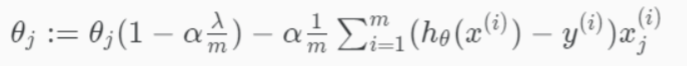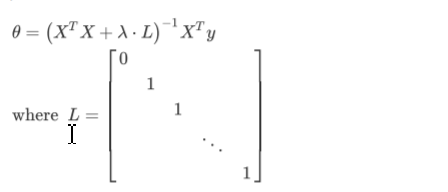• Regularized Logistic Regression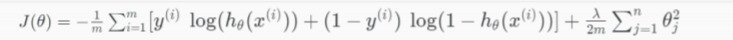### Neural Networks

• Model Representation

• Forward propagation:Vectorized implementation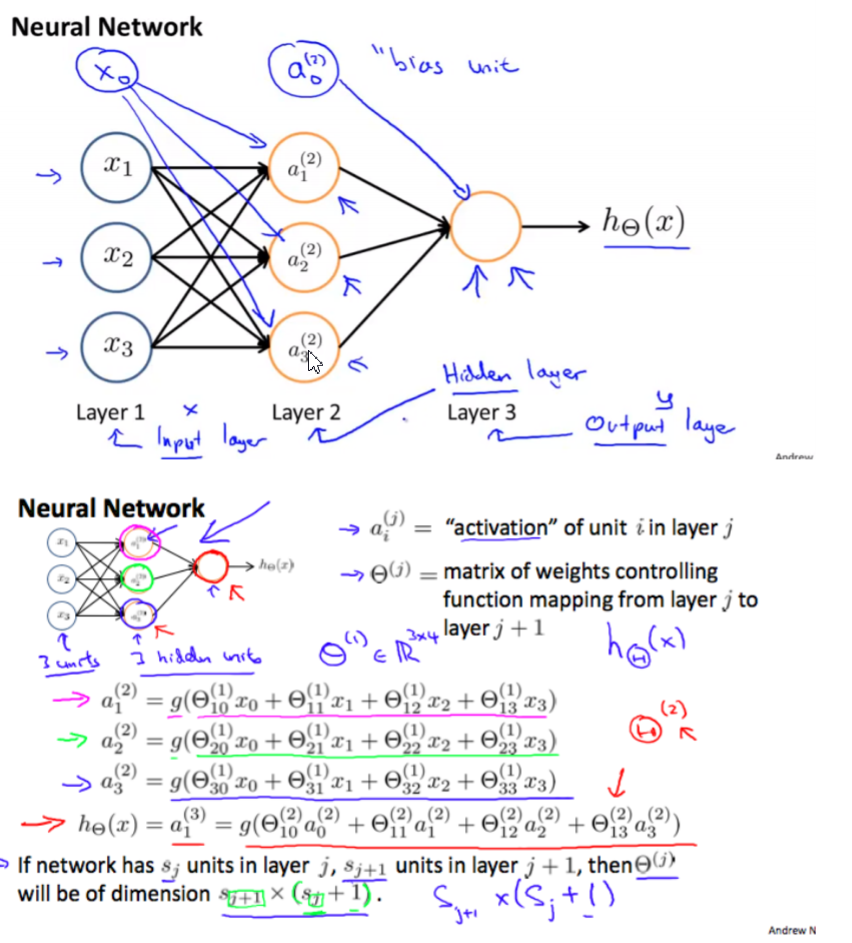• Multiclass Classification

one-vs-all• Neural Network(Classification)

L = total number of layers in the network
$s_l$= number of units (not counting bias unit) in layer l
K = number of output units/classes

• Cost Function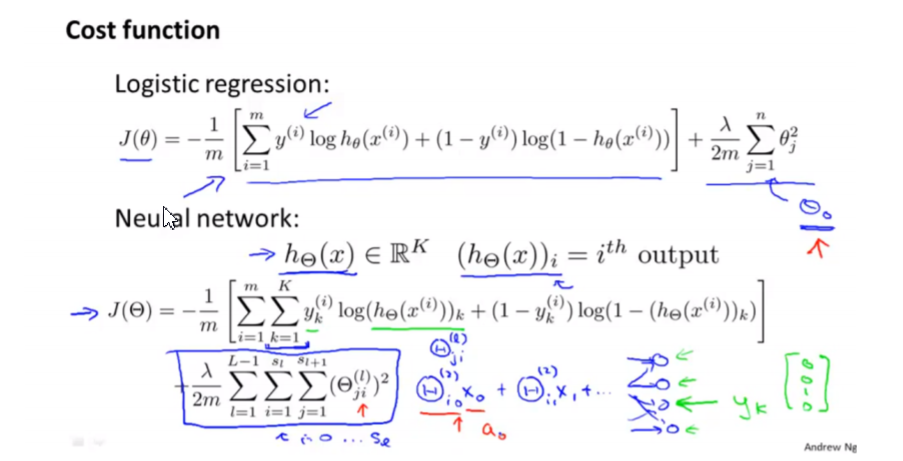• Backpropagation Algorithm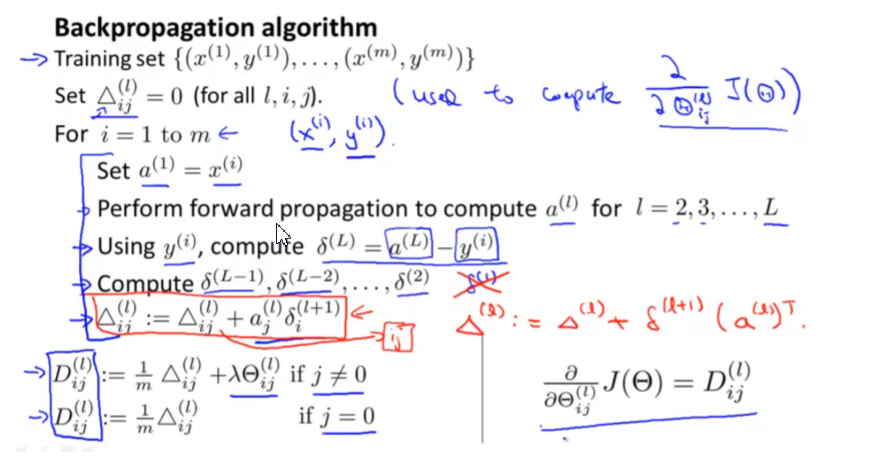• Gradient Checking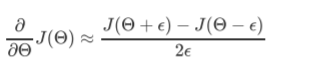• Random Initialization